# Graphics Final Project

## Photon Simulation

### Kyle Brunnett - CPE 471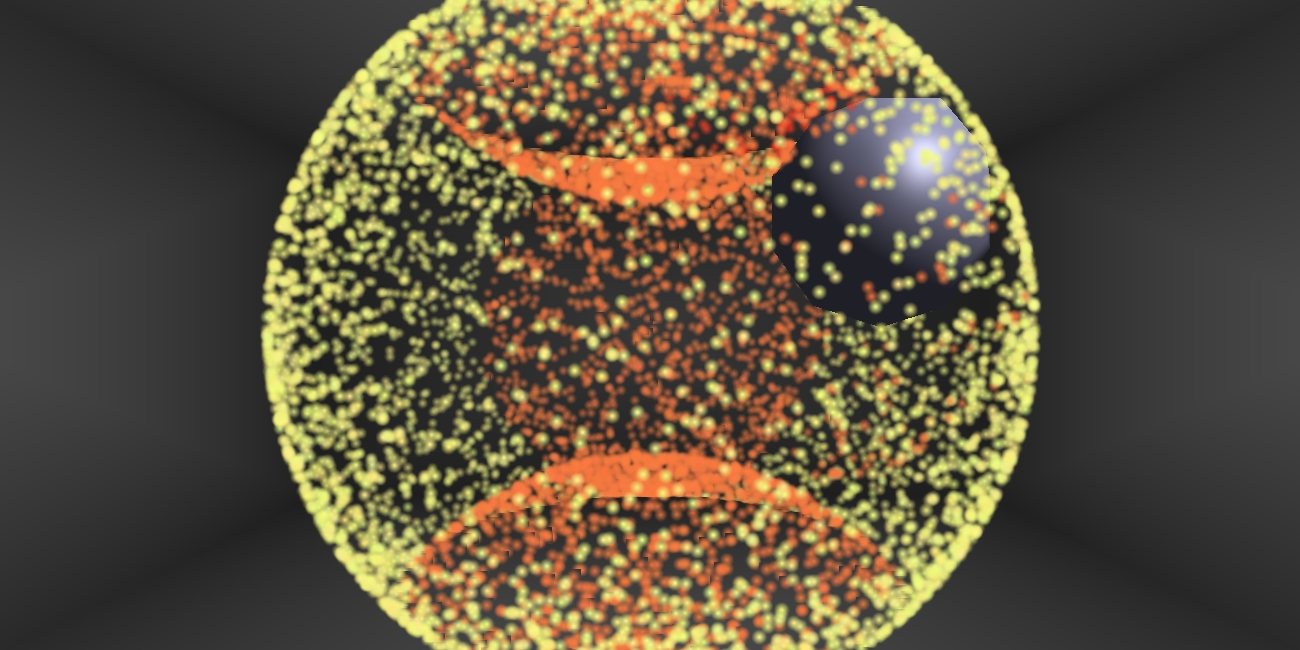### Introduction

The purpose of this project was to simulate the interaction of thousands of photons in a small room with various movable objects. The photons are all emitted from the same position, with random velocities of the same speed. Below, you can see a simulation of 5000 of these particles, emitted in a small sector toward an object in order to maximize their density.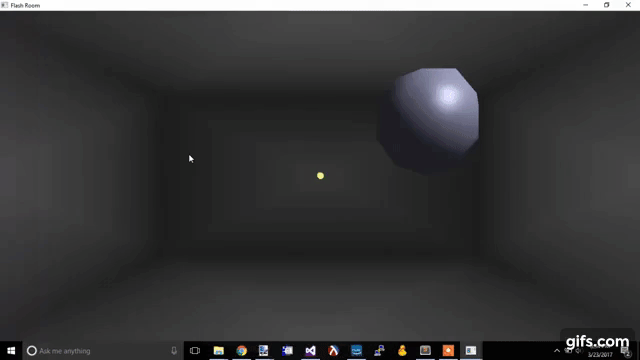The photons in the scene are spawned as glowing photons of a random yellow color (specifically [0.95, 0.95, 0.5] ± .05 in each component) Originally, these photons remained the same color throughout their lives, but this webpage displays a modified version that approach full red (1.0, 0.0, 0.0) with each collision, to make the static images easier to view.
In order to give the photons a preception of being 3D, the photons get more transparent (i.e. less "intense") and smaller as they get farther from the camera's position.

### User Interaction

The user can interact with the simulation using the following keys:

space bar: restore the scene to its default state

left mouse click: spawn the photons at the current cursor position

right mouse click: move the sphere to the current cursor position

X, Y, Z keys: move the sphere along the possitive x, y, and z axes.

All positions are determined to be on the edge of the rooms, since the user has no obvious way of communicating the depth of a click.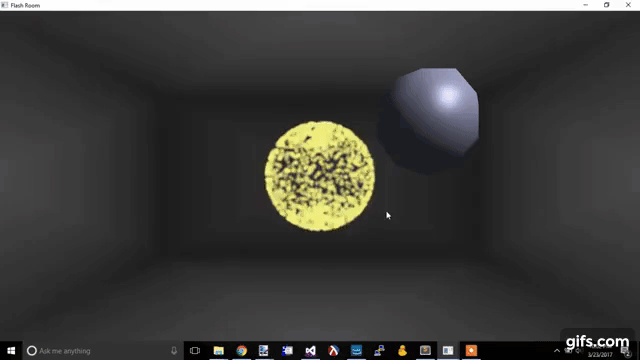### Configuration

The program has two constants that configure the scene at compile-time:

NUM_PHOTONS: The number of photons that are on the screen at a time. As this affects the update frequency of the program, the speed decays exponentially with higher number of photons.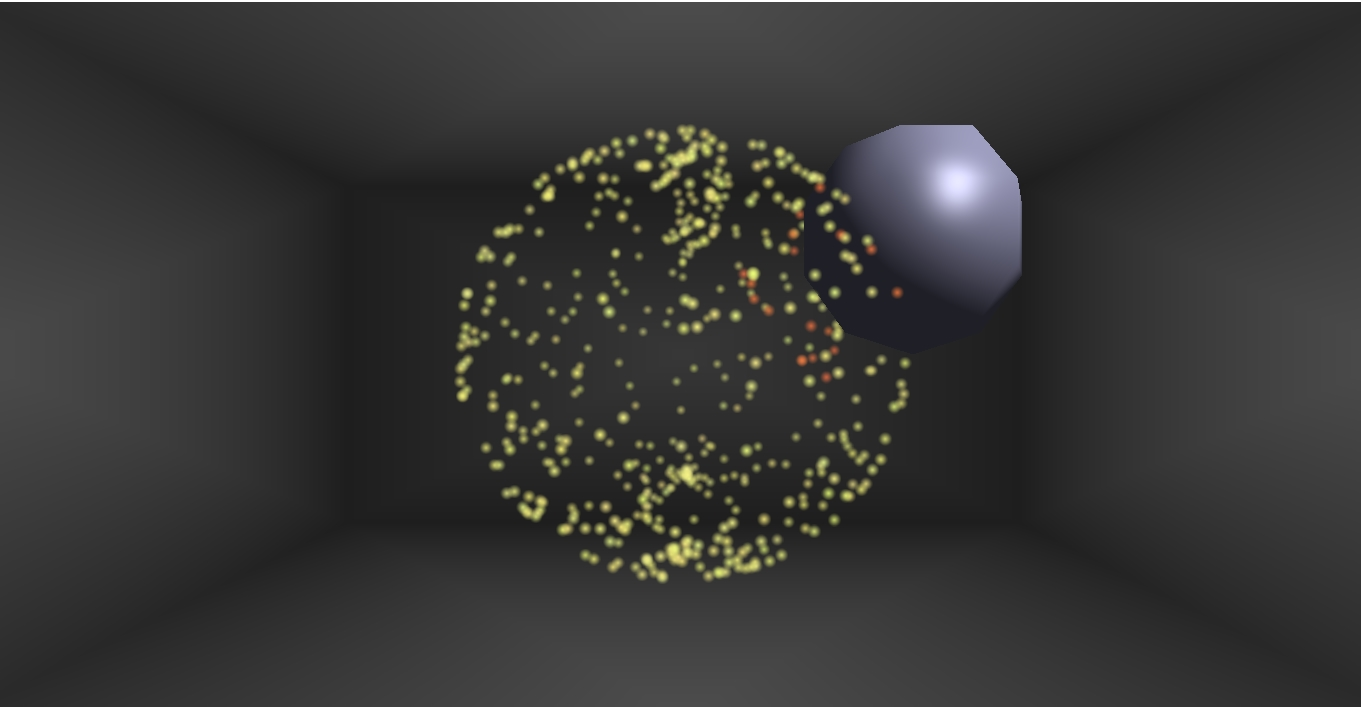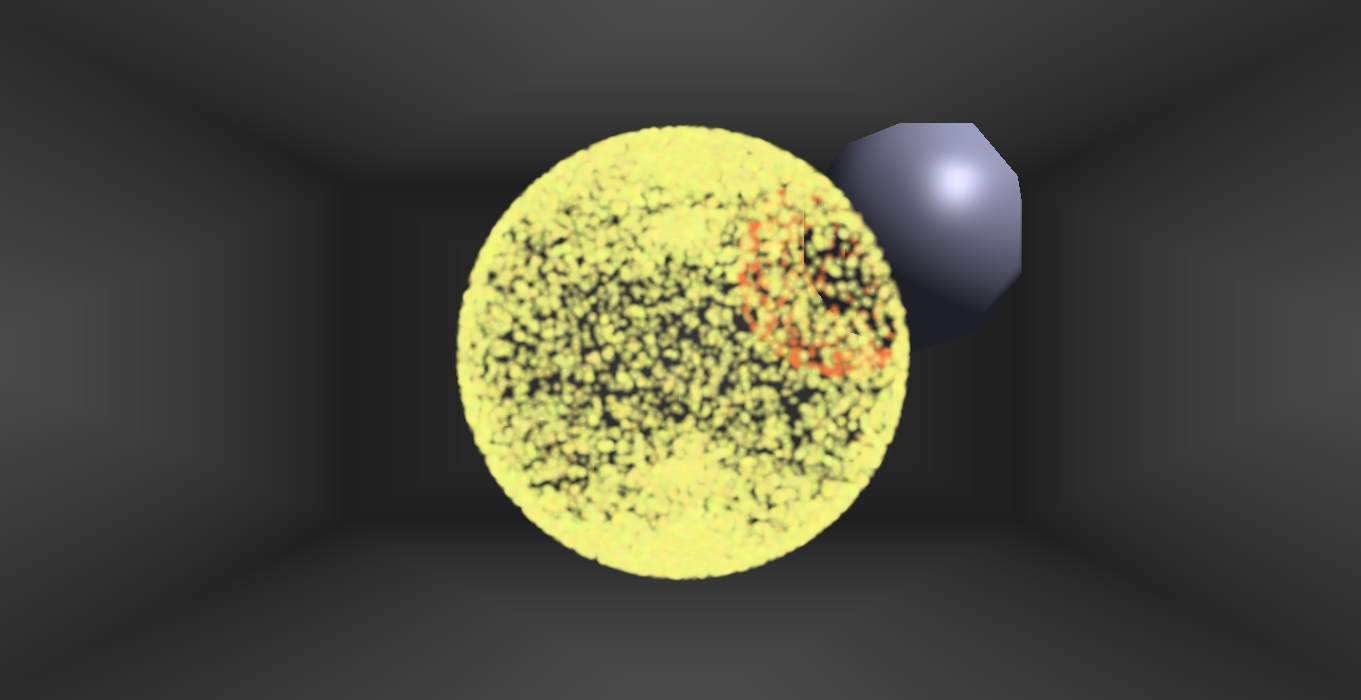SPHERE: Determines the shape of the particle emission. A value of 0 represents a small sector of photons emitted toward the default object position, while a value of 1 represents a sphere growing in every direction.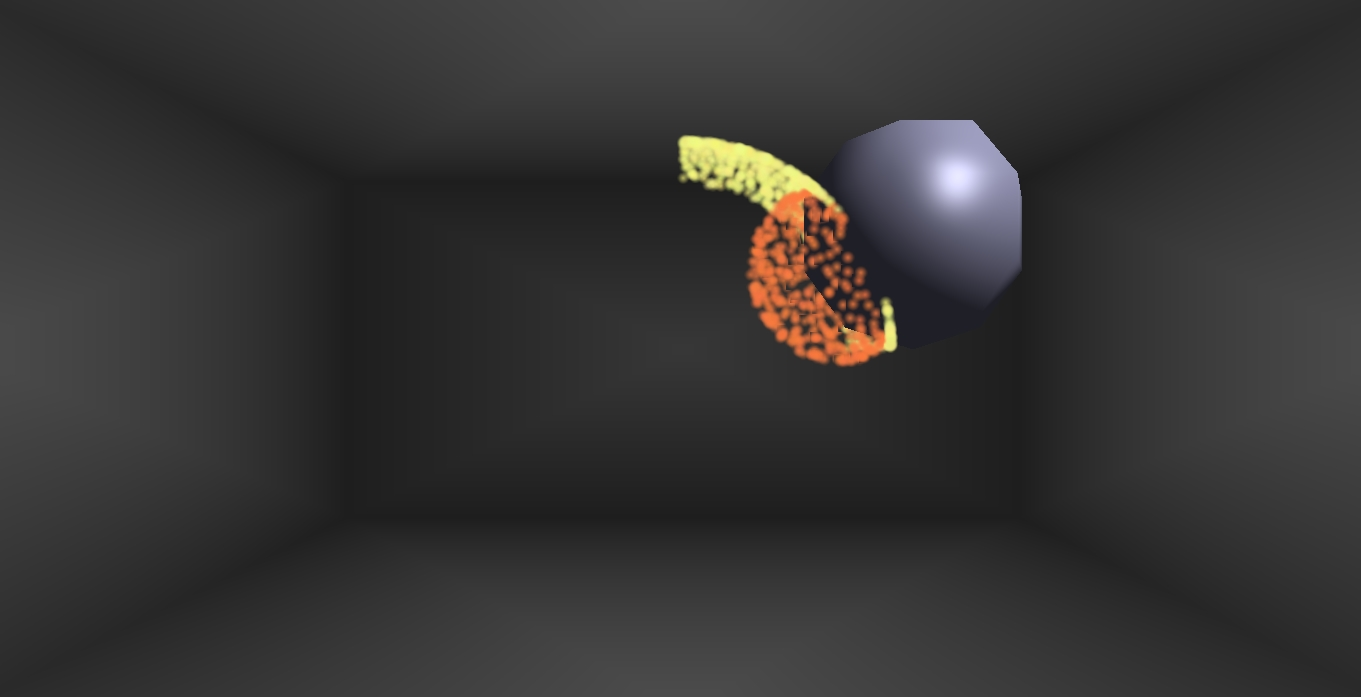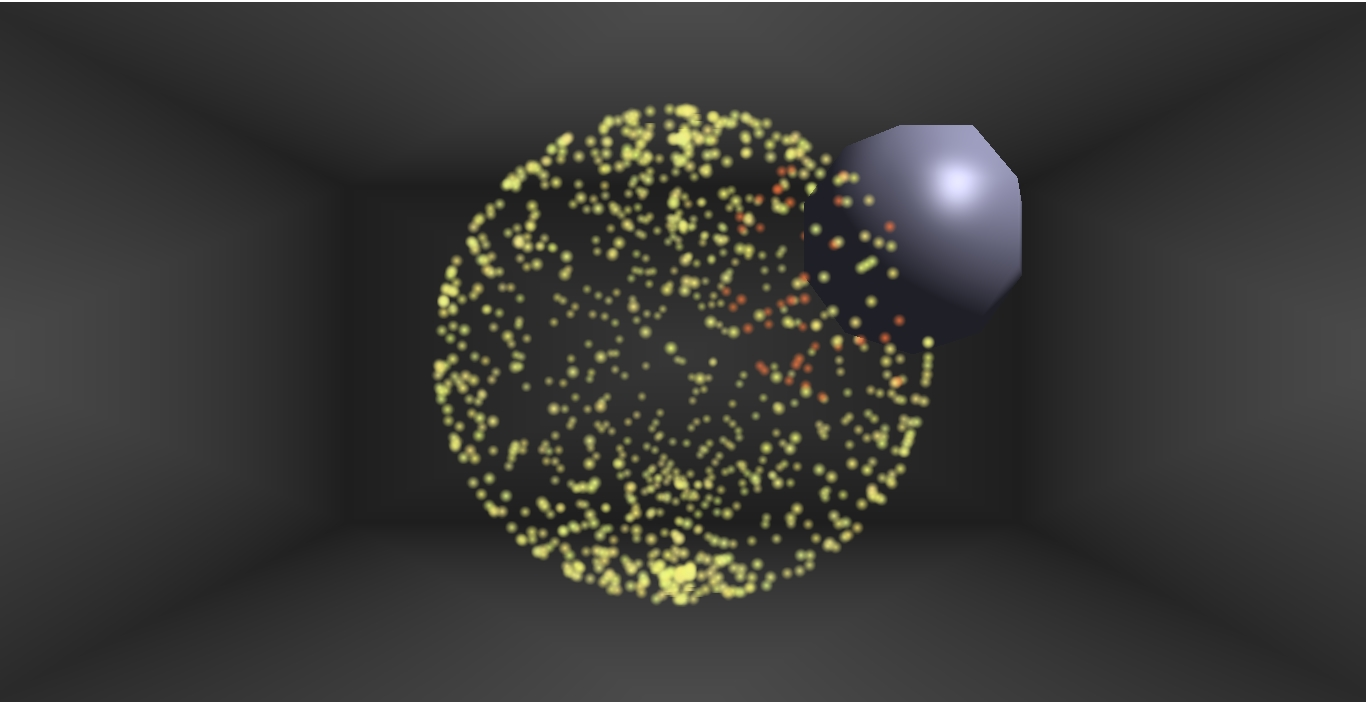### Collision Simulation

There are two types of collisions that occur in the scene.

The first is between the photons and the walls, which are achieved by checking each photon particle's x, y, and z components against a fixed set of values, since the room size does not change. The planes that form the floor, walls, and ceiling are placed at these offsets. If the particle lies outside one of the planes, the appropriate component of its velocity is reversed, reflecting the photon as it would in the real world.

The second, and more interesting, type of collision is between the photons and the sphere. These collisions are simulated by first checking the distance of each photon from the point at the center of the sphere, passed as an argument to each particle's update() function. If a collision is detected, then the normal is computed by subtracting the sphere's center from the photon's position (N = Photon - Sphere). The reflection vector is then calculated using the equation R = speed * ( -V + 2 * N · -V) * N), where V and N are normalized velocity and normal vectors, respectively.

### Conclusion

While this simulation is accurate for what it is able to display, the performance and capability would be much more extensive if all calculations were ported to the shaders to be performed on the GPU. The gratest weakness of this program is that most of the computational work is done in serial on the CPU, slowing the simulation down for just a few thousand particles and a few collision detections. Parallelizing the velocity updates and collision detection tests would allow for magnitudes more particles, opening up the possibility of finer-grained representation of photons. It would also potentially open the door for collisions with more complex, non-spherical objects, which was the original goal: a real-time simulation of the interaction between photons and normal .obj representations of objects.